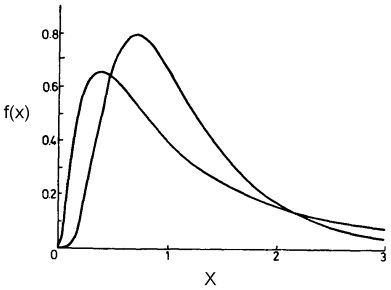Join one of our upcoming courses taking place around the world! Explore now

# Lognormal Distribution

If a random variable has a Lognormal Distribution, its natural logarithm has a Normal Distribution. This is the meaning of the term Lognormal.

Like the Exponential Distribution, the Lognormal Distribution can only be used for variables that are always positive.

A Lognormal Distribution can be useful for modeling variables such as cohesion, for example, which may have a large peak in the distribution near zero and then taper off gradually for larger values.Lognormal probability density functions